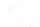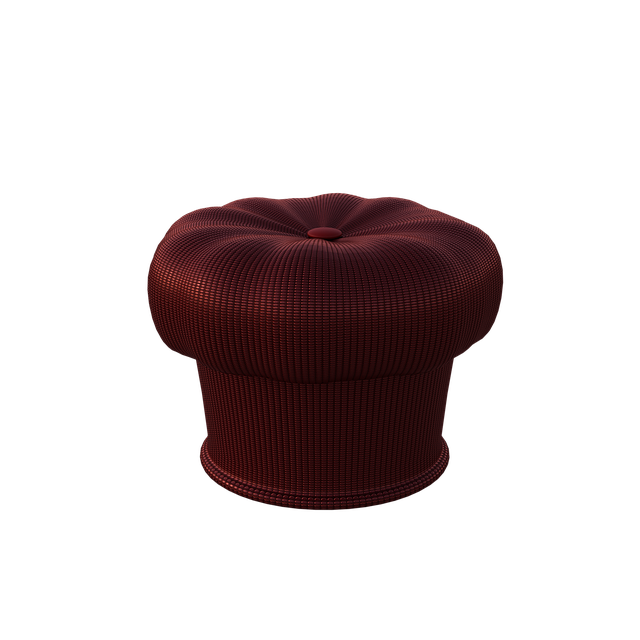# //<![CDATA[ (function(){for(var g="function"==typeof Object.defineProperties?Object.defineProperty:function(b,c,a){if(a.get||a.set)throw new TypeError("ES3 does not support getters and setters.");b!=Array.prototype&&b!=Object.prototype&&(b[c]=a.value)},h="undefined"!=typeof window&&window===this?this:"undefined"!=typeof global&&null!=global?global:this,k=["String","prototype","repeat"],l=0;l<k.length-1;l++){var m=k[l];m in h||(h[m]={});h=h[m]}var n=k[k.length-1],p=h[n],q=p?p:function(b){var c;if(null==this)throw new TypeError("The 'this' value for String.prototype.repeat must not be null or undefined");c=this+"";if(0>b||1342177279<b)throw new RangeError("Invalid count value");b|=0;for(var a="";b;)if(b&1&&(a+=c),b>>>=1)c+=c;return a};q!=p&&null!=q&&g(h,n,{configurable:!0,writable:!0,value:q});var t=this;function u(b,c){var a=b.split("."),d=t;ain d||!d.execScript||d.execScript("var "+a);for(var e;a.length&&(e=a.shift());)a.length||void 0===c?d[e]?d=d[e]:d=d[e]={}:d[e]=c};function v(b){var c=b.length;if(0<c){for(var a=Array(c),d=0;d<c;d++)a[d]=b[d];return a}return[]};function w(b){var c=window;if(c.addEventListener)c.addEventListener("load",b,!1);else if(c.attachEvent)c.attachEvent("onload",b);else{var a=c.onload;c.onload=function(){b.call(this);a&&a.call(this)}}};var x;function y(b,c,a,d,e){this.h=b;this.j=c;this.l=a;this.f=e;this.g={height:window.innerHeight||document.documentElement.clientHeight||document.body.clientHeight,width:window.innerWidth||document.documentElement.clientWidth||document.body.clientWidth};this.i=d;this.b={};this.a=[];this.c={}}function z(b,c){var a,d,e=c.getAttribute("data-pagespeed-url-hash");if(a=e&&!(e in b.c))if(0>=c.offsetWidth&&0>=c.offsetHeight)a=!1;else{d=c.getBoundingClientRect();var f=document.body;a=d.top+("pageYOffset"in window?window.pageYOffset:(document.documentElement||f.parentNode||f).scrollTop);d=d.left+("pageXOffset"in window?window.pageXOffset:(document.documentElement||f.parentNode||f).scrollLeft);f=a.toString()+","+d;b.b.hasOwnProperty(f)?a=!1:(b.b[f]=!0,a=a<=b.g.height&&d<=b.g.width)}a&&(b.a.push(e),b.c[e]=!0)}y.prototype.checkImageForCriticality=function(b){b.getBoundingClientRect&&z(this,b)};u("pagespeed.CriticalImages.checkImageForCriticality",function(b){x.checkImageForCriticality(b)});u("pagespeed.CriticalImages.checkCriticalImages",function(){A(x)});function A(b){b.b={};for(var c=["IMG","INPUT"],a=[],d=0;d<c.length;++d)a=a.concat(v(document.getElementsByTagName(c[d])));if(a.length&&a.getBoundingClientRect){for(d=0;c=a[d];++d)z(b,c);a="oh="+b.l;b.f&&(a+="&n="+b.f);if(c=!!b.a.length)for(a+="&ci="+encodeURIComponent(b.a),d=1;d<b.a.length;++d){var e=","+encodeURIComponent(b.a[d]);131072>=a.length+e.length&&(a+=e)}b.i&&(e="&rd="+encodeURIComponent(JSON.stringify(B())),131072>=a.length+e.length&&(a+=e),c=!0);C=a;if(c){d=b.h;b=b.j;var f;if(window.XMLHttpRequest)f=new XMLHttpRequest;else if(window.ActiveXObject)try{f=new ActiveXObject("Msxml2.XMLHTTP")}catch(r){try{f=new ActiveXObject("Microsoft.XMLHTTP")}catch(D){}}f&&(f.open("POST",d+(-1==d.indexOf("?")?"?":"&")+"url="+encodeURIComponent(b)),f.setRequestHeader("Content-Type","application/x-www-form-urlencoded"),f.send(a))}}}function B(){var b={},c;c=document.getElementsByTagName("IMG");if(!c.length)return{};var a=c;if(!("naturalWidth"in a&&"naturalHeight"in a))return{};for(var d=0;a=c[d];++d){var e=a.getAttribute("data-pagespeed-url-hash");e&&(!(e in b)&&0<a.width&&0<a.height&&0<a.naturalWidth&&0<a.naturalHeight||e in b&&a.width>=b[e].o&&a.height>=b[e].m)&&(b[e]={rw:a.width,rh:a.height,ow:a.naturalWidth,oh:a.naturalHeight})}return b}var C="";u("pagespeed.CriticalImages.getBeaconData",function(){return C});u("pagespeed.CriticalImages.Run",function(b,c,a,d,e,f){var r=new y(b,c,a,e,f);x=r;d&&w(function(){window.setTimeout(function(){A(r)},0)})});})();pagespeed.CriticalImages.Run('/mod_pagespeed_beacon','https://www.offidocs.com/index.php/main-templates/illustrations-examples/4754-chair-seat-fabric','mPY1vkVZCX',true,false,'vL1lrujaGps'); //]]>## Chair Seat FabricThis is the illustration named Chair Seat Fabric for OffiDocs app Gimp online image or photo editor

### TAGS:

Download or edit the template Chair Seat Fabric for GIMP online editor.

chair, seat, fabric, furniture, interior, sit, decor, style, modern, design, indoors, comfortable, decoration, funny, seating, relax, Free illustrations, Free Images.

Free download template Chair Seat Fabric integrated with the OffiDocs web apps